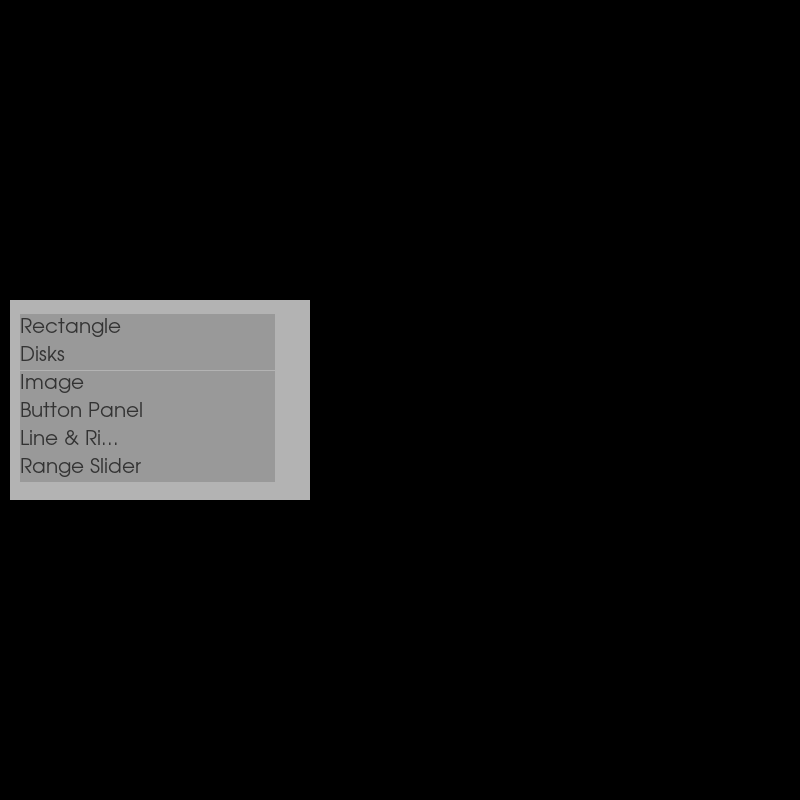# User Interfaces¶

This example shows how to use the UI API. We will demonstrate how to create several DIPY UI elements, then use a list box to toggle which element is shown.

First, a bunch of imports.

from fury.data import read_viz_icons, fetch_viz_icons

from fury import ui, window


## Shapes¶

Let’s start by drawing some simple shapes. First, a rectangle.

rect = ui.Rectangle2D(size=(200, 200), position=(400, 300), color=(1, 0, 1))


Then we can draw a solid circle, or disk.

disk = ui.Disk2D(outer_radius=50, center=(500, 500), color=(1, 1, 0))


Add an inner radius to make a ring.

ring = ui.Disk2D(outer_radius=50, inner_radius=45, center=(500, 300),
color=(0, 1, 1))


## Image¶

Now let’s display an image. First we need to fetch some icons that are included in DIPY.

fetch_viz_icons()


Out:

Data size is approximately 12KB
Dataset is already in place. If you want to fetch it again please first remove the folder /Users/koudoro/.fury/icons


Now we can create an image container.

img = ui.ImageContainer2D(img_path=read_viz_icons(fname='home3.png'),
position=(450, 350))


## Panel with buttons and text¶

Let’s create some buttons and text and put them in a panel. First we’ll make the panel.

panel = ui.Panel2D(size=(300, 150), color=(1, 1, 1), align="right")
panel.center = (500, 400)


Then we’ll make two text labels and place them on the panel. Note that we specifiy the position with integer numbers of pixels.

text = ui.TextBlock2D(text='Click me')
text2 = ui.TextBlock2D(text='Me too')


Then we’ll create two buttons and add them to the panel.

Note that here we specify the positions with floats. In this case, these are percentages of the panel size.

button_example = ui.Button2D(

icon_files = []

second_button_example = ui.Button2D(icon_fnames=icon_files)



We can add a callback to each button to perform some action.

def change_text_callback(i_ren, _obj, _button):
text.message = 'Clicked!'
i_ren.force_render()

def change_icon_callback(i_ren, _obj, _button):
button.next_icon()
i_ren.force_render()

button_example.on_left_mouse_button_clicked = change_text_callback
second_button_example.on_left_mouse_button_pressed = change_icon_callback


## Cube and sliders¶

Let’s add a cube to the scene and control it with sliders.

def cube_maker(color=(1, 1, 1), size=(0.2, 0.2, 0.2), center=(0, 0, 0)):
cube = window.vtk.vtkCubeSource()
cube.SetXLength(size)
cube.SetYLength(size)
cube.SetZLength(size)
if center is not None:
cube.SetCenter(*center)
cube_mapper = window.vtk.vtkPolyDataMapper()
cube_mapper.SetInputConnection(cube.GetOutputPort())
cube_actor = window.vtk.vtkActor()
cube_actor.SetMapper(cube_mapper)
if color is not None:
cube_actor.GetProperty().SetColor(color)
return cube_actor

cube = cube_maker(color=(0, 0, 1), size=(20, 20, 20), center=(15, 0, 0))


Now we’ll add two sliders: one circular and one linear.

ring_slider = ui.RingSlider2D(center=(740, 400), initial_value=0,
text_template="{angle:5.1f}°")

line_slider = ui.LineSlider2D(center=(500, 250), initial_value=0,
min_value=-10, max_value=10)


We can use a callback to rotate the cube with the ring slider.

def rotate_cube(slider):
angle = slider.value
previous_angle = slider.previous_value
rotation_angle = angle - previous_angle
cube.RotateX(rotation_angle)

ring_slider.on_change = rotate_cube


Similarly, we can translate the cube with the line slider.

def translate_cube(slider):
value = slider.value
cube.SetPosition(value, 0, 0)

line_slider.on_change = translate_cube


## Range Slider¶

Finally, we can add a range slider. This element is composed of two sliders. The first slider has two handles which let you set the range of the second.

range_slider = ui.RangeSlider(
line_width=8, handle_side=25, range_slider_center=(550, 450),
value_slider_center=(550, 350), length=250, min_value=0,
max_value=10, font_size=18, range_precision=2, value_precision=4,
shape="square")


We just added many examples. If we showed them all at once, they would fill the screen. Let’s make a simple menu to choose which example is shown.

We’ll first make a list of the examples.

examples = [[rect], [disk, ring], [img], [panel],
[ring_slider, line_slider], [range_slider]]


Now we’ll make a function to hide all the examples. Then we’ll call it so that none are shown initially.

def hide_all_examples():
for example in examples:
for element in example:
element.set_visibility(False)
cube.SetVisibility(False)

hide_all_examples()


To make the menu, we’ll first need to create a list of labels which correspond with the examples.

values = ['Rectangle', 'Disks', 'Image', "Button Panel",
"Line and Ring Slider", "Range Slider"]


Now we can create the menu.

listbox = ui.ListBox2D(values=values, position=(10, 300), size=(300, 200),
multiselection=False)


Then we will use a callback to show the correct example when a label is clicked.

def display_element():
hide_all_examples()
example = examples[values.index(listbox.selected)]
for element in example:
element.set_visibility(True)
if values.index(listbox.selected) == 4:
cube.SetVisibility(True)

listbox.on_change = display_element


## Show Manager¶

Now that all the elements have been initialised, we add them to the show manager.

current_size = (800, 800)
show_manager = window.ShowManager(size=current_size, title="DIPY UI Example")

for example in examples:
for element in example:
show_manager.scene.reset_camera()
show_manager.scene.set_camera(position=(0, 0, 200))
show_manager.scene.reset_clipping_range()
show_manager.scene.azimuth(30)

interactive = False

if interactive:
show_manager.start()

window.record(show_manager.scene, size=current_size, out_path="viz_ui.png")Total running time of the script: ( 0 minutes 0.156 seconds)

Gallery generated by Sphinx-Gallery